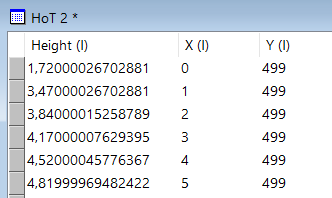Home - General / All posts - Calculating Height from raster file derived from LiDAR scan
 yves61153 post(s) #13-Sep-18 12:54 I have :a) "DSM_1m" a (tif) raster file derived from LiDAR scans that I imported in my project map.I copied DSM_1m and pasted it as a table : DSM_1m_tbl. Height in this table reflects the height of any construction or other object (tree, ...) on top of the ground level.b) "DTM_1m" a (tif) raster file derived from LiDAR scans that I imported in my project map.I copied DTM_1m and pasted it as a table : DTM_1m_tbl. Height in this table reflects atr ground level.Both DSM and DTM rasters have a resolution of 1 pixel equals 1 m.The points in DSM and DTM coincide as to their location. Substracting the height value in the DSM from the height value in the DTM yields the height of the building above ground level at the given point.c) Building drawings is self-explanatory.Can someone help me with this - so basic query - in order to calculate the volume of each individual building area and add the volume "value" to a still to be added volume "field" in the Buildings Drawing table.
 yves61153 post(s) #13-Sep-18 13:01 I want to upload my project map as an example but this seems not to work well. Is it due to the size of the map ? 21 MB.
 vincent1,774 post(s) #13-Sep-18 13:20 Pasting as a table is not necessary. Do you have Surface Tools ? It gives you more options to play with raster.In the Surface top menu, there is "Transform". You can use it to subtract A-B to obtain height over ground.You can use SQL as well. See "Raster extensions". You can use SQL to obtain volume. Volume(surface name, geom).That would give someting like :Update [Building Drawing] Set [volume_field] = Volume([height_above_ground_raster], [Geom (I)]).
 yves61153 post(s) #13-Sep-18 14:25 Vincent; Thank you for your help.I have Surface Tools and I managed to use the Transform tool to substract DSM_1m from DTM_1m and saved the result in a new component "HoT".With SQL I can use Volume(surface name, geom):surface name = "HoT", but as to geom in Volume(surface name, geom) I do not know the syntaxis to use.I suppose I can use X(I), Y(I). But what would be the exact syntaxis ?Can you help any further ?
 vincent1,774 post(s) #13-Sep-18 14:52 Did you try with [Geom (I)] ? That's an intrinsect field (like all the (I)).This field is from the drawing.
 yves61153 post(s) #13-Sep-18 15:02 modifying my reply...Vincent, I attach my project map to clarify things.So I have now a Buildings drawing with areas, and I have a surface component called "HoT" which is the result of subtracting DSM_1m and DTM_1m as explained earlier.I understand thata) for each individual building "area" I need to select the pixels contained within each building area ,b) calculate the average value of the heights of the contained pixels,c) than multiply the average height with the area of the building itemsWhat would the SQL code be ?Many thanks in advance.
 yves61153 post(s) #13-Sep-18 15:29 I expect the SQL code must be likeselect [Buildings Drawing].[ID], [Buildings Drawing].[Area (I)], [HoT].[Height (I)],[HoT].[Center Easting (I)],[HoT].[Center Northing (I)],Avg ([Hot].[Height (I)]) as AvH,[Buildings Drawing].[Area (I)] * Avg ([Hot].[Height (I)])FROM [Buildings Drawing], [HoT] where Contains ([Buildings Drawing].[ID], ??????? )group by [Buildings Drawing].[ID]-- what to put in place of ???? , the geom for each pixel coordinate in [Hot], What is the right syntaxis ?Thank you in advance for guiding.
 vincent1,774 post(s) #13-Sep-18 15:50 Assuming you have a drawing "Buildings" with multiples buildings in it, with a field "volume". Run this query : Update [Buildings] SET [volume] = Volume ([HoT], [Geom (I)])It calculates the volume for each pixels inside buildings and returns the sum in the "volume" field for each building. Too simple ?
 vincent1,774 post(s) #13-Sep-18 15:52 "The function assumes surface heights are in meters, and returns the volume in cubic meters. To compute volume, the system takes all pixels, computes the volume of each pixel multiplying its height (which can be negative) by its area, and sums the resulting volumes. Hence, the volume can be less than zero"
 yves61153 post(s) #13-Sep-18 16:15 Vincent, thank you very much. Really this was too simple ! Did not understand the function Volume() well. I thought I needed to select pixels contained within the building areas ... etcetera...Thanks again. Works perfect !